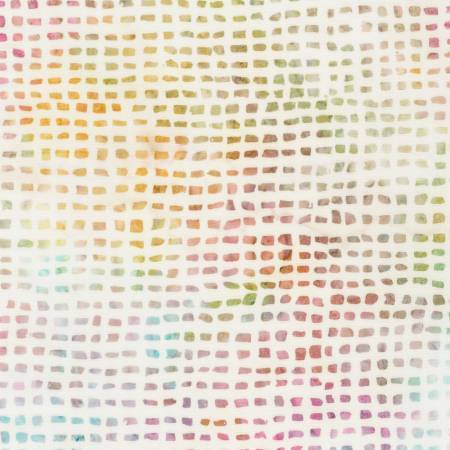this one is my fav - kinda different!

# Who Loves Batiks?

Then you are going to be a happy shopper, because we received a big shipment of Batiks in blues, greens, purples and corals - all the colors you love!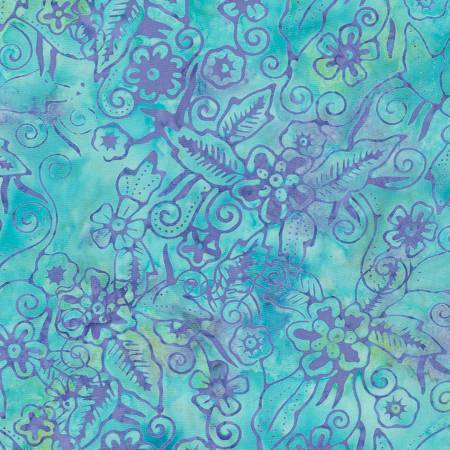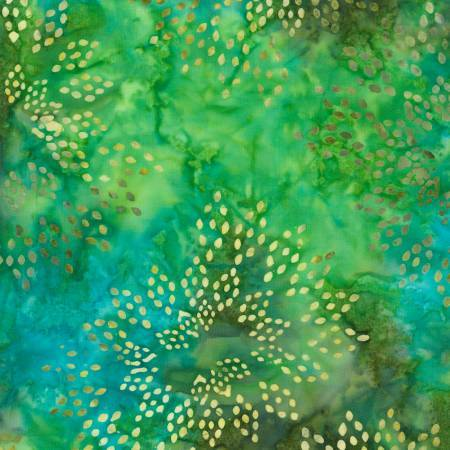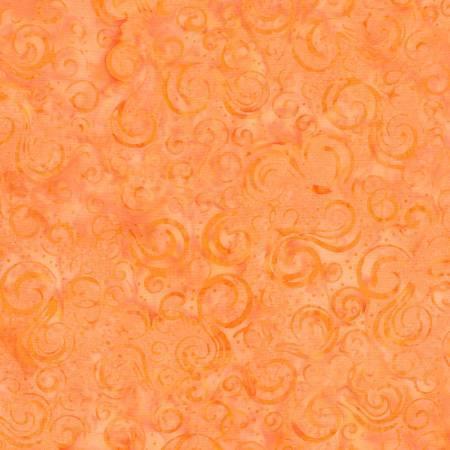table div table+table+table+table+table div table{width:100%;padding:0}table div table+table+table+table+table div table img{width:96.23%;padding:0;float:none}table div table+table+table+table+table div table td{width:100%;padding:0 1.88% 18px}/* styles */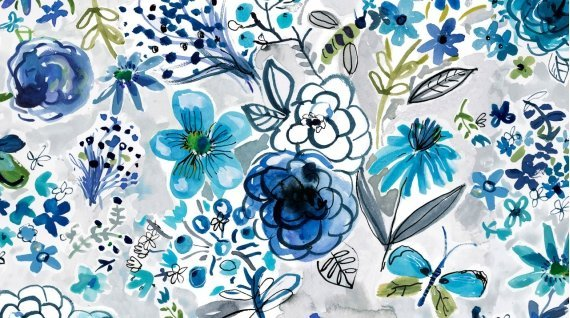# Blue Crush

Crushing on this Blue Crush!
August Wren is a new designer for Dear Stella - these are digitally printed but they look like they are painted!
The birds, the cottages, the florals and a great painterly stripe!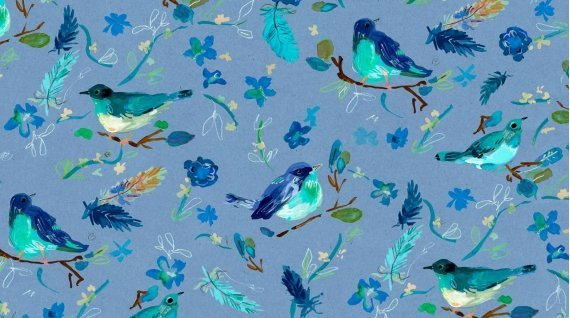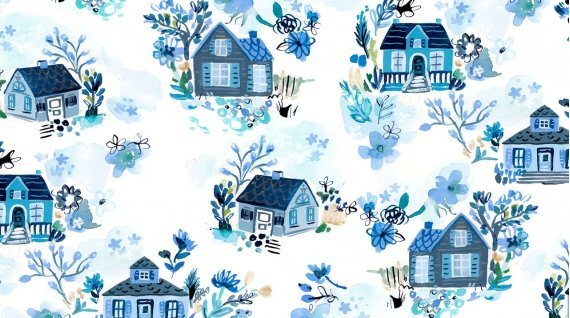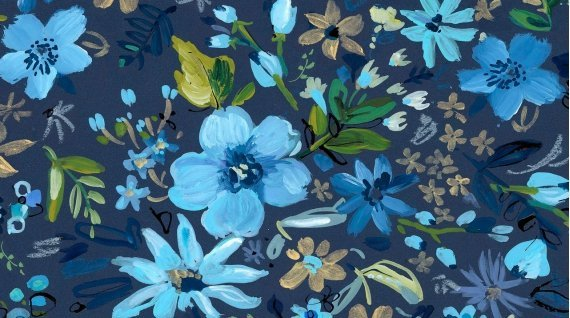table div table+table+table+table+table+table+table+table+table div table{width:100%;padding:0}table div table+table+table+table+table+table+table+table+table div table img{width:96.23%;padding:0;float:none}table div table+table+table+table+table+table+table+table+table div table td{width:100%;padding:0 1.88% 18px}/* styles */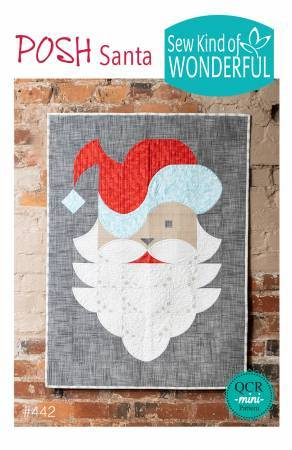# New Books and Patterns!

Two new patterns using the Quick Curve Rulers
New Book from Terry Atkinson
Malibu Sling
and more!

 table div table+table+table+table+table+table+table+table+table+table+table+table div table{width:100%;padding:0}table div table+table+table+table+table+table+table+table+table+table+table+table div table img{width:96.23%;padding:0;float:none}table div table+table+table+table+table+table+table+table+table+table+table+table div table td{width:100%;padding:0 1.88% 18px}/* styles */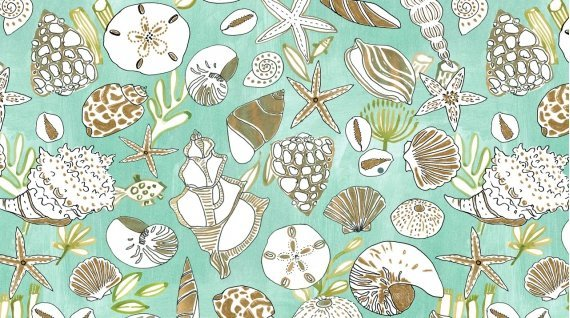# Lots of New Goodies!

The new issue of Quilt Sampler is here - take a look at 10 Top Shops and their exclusive projects!
Sewing themed greeting cards!
New Square Bobbin Saver
Shell and Mermaid fabric
Be sure to check them all out!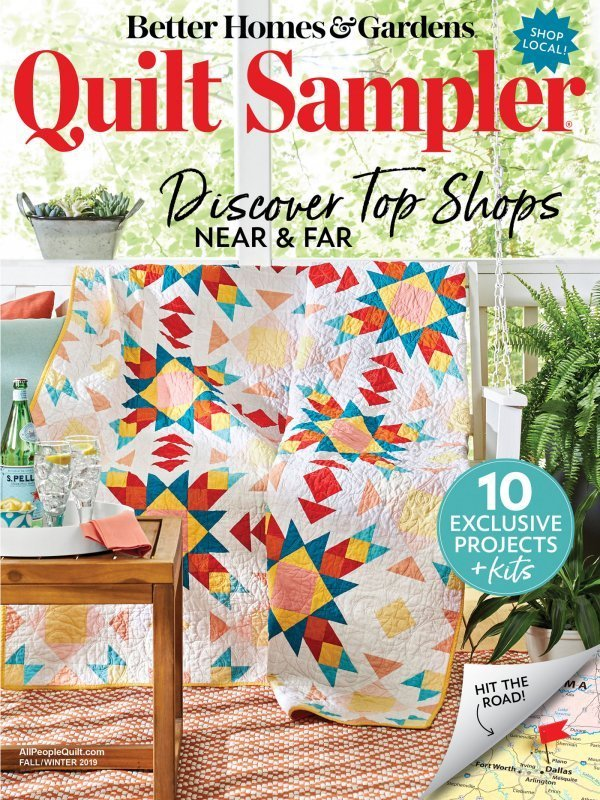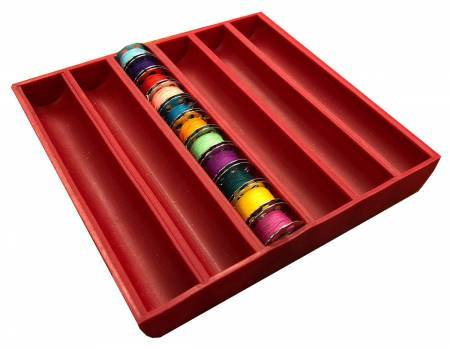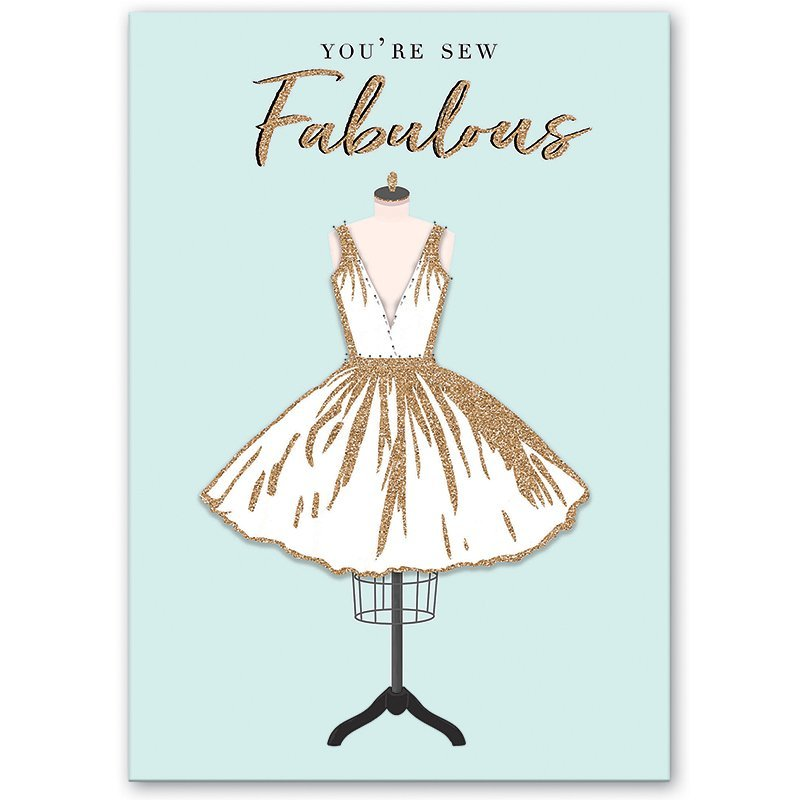table div table+table+table+table+table+table+table+table+table+table+table+table+table+table+table+table div table{width:100%;padding:0}table div table+table+table+table+table+table+table+table+table+table+table+table+table+table+table+table div table img{width:96.23%;padding:0;float:none}table div table+table+table+table+table+table+table+table+table+table+table+table+table+table+table+table div table td{width:100%;padding:0 1.88% 18px}/* styles */## And, here's a little secret,

these brand new arrivals were revealed for the first time on our Facebook Live Sunday Soiree along with special pricing for that night only,
so if you like being first in-the-know,
tune in on Sunday evenings at 7pm eastern time!

 table div table+table+table+table+table+table+table+table+table+table+table+table+table+table+table+table+table+table div table{width:100%;padding:0}table div table+table+table+table+table+table+table+table+table+table+table+table+table+table+table+table+table+table div table img{width:96.23%;padding:0;float:none}table div table+table+table+table+table+table+table+table+table+table+table+table+table+table+table+table+table+table div table td{width:100%;padding:0 1.88% 18px}/* styles */# Next PMS Night is Friday, September 13

6pm to midnight
Treat yourself to a night of food, fabric and friends!
Who knows, you could be the next fat quarter winner!

 table div table+table+table+table+table+table+table+table+table+table+table+table+table+table+table+table+table+table+table+table+table div table{width:100%;padding:0}table div table+table+table+table+table+table+table+table+table+table+table+table+table+table+table+table+table+table+table+table+table div table img{width:96.23%;padding:0;float:none}table div table+table+table+table+table+table+table+table+table+table+table+table+table+table+table+table+table+table+table+table+table div table td{width:100%;padding:0 1.88% 18px}/* styles */# Casual Sew 10am to 4pm

Friday September 6, 13, 30, 27

# Upcoming Classes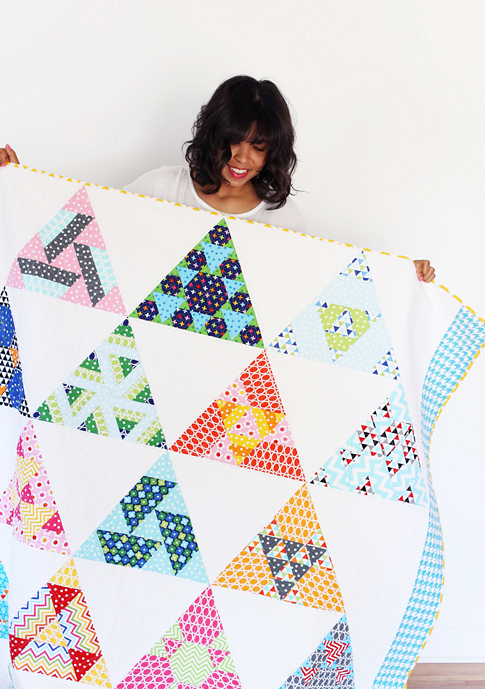# Toes in the Sand

It's not too late to join in the fun!
Class meets 6 more times.
Next class is Saturday, September 7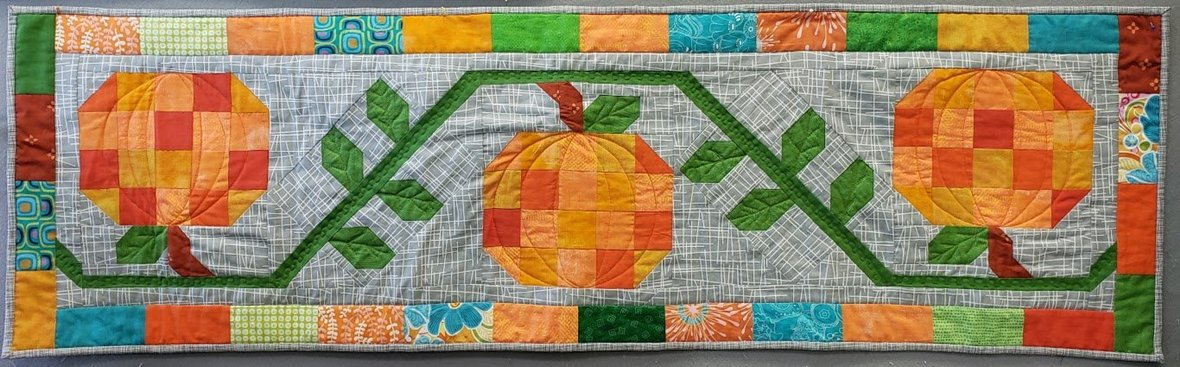# Pumpkin Patch Table Runner

Dig out your orange fabrics and make this darling table runner!
Class is Sunday, September 8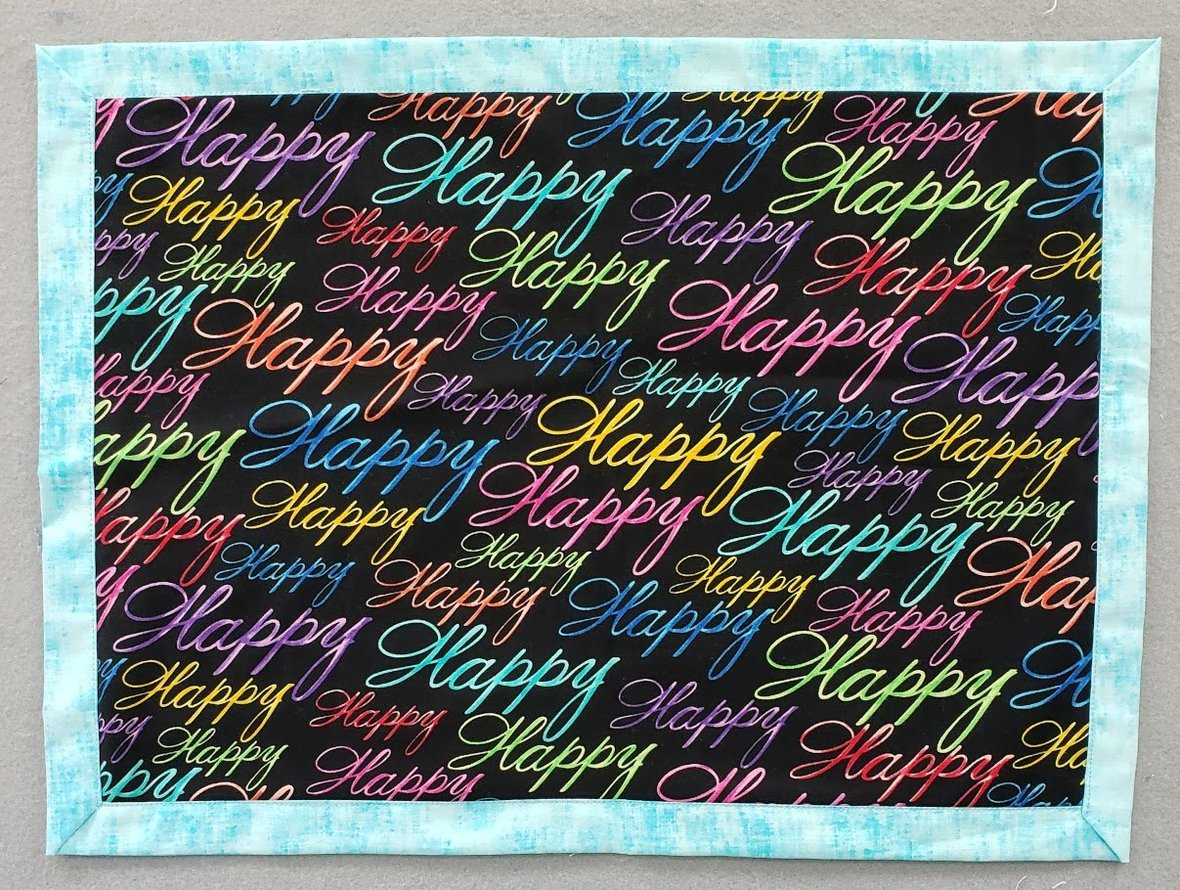# SewDays are coming!

==========
September 9, 16 and 23 we are having #SewDays at Cotton Patch!
3 easy projects chosen with the new/inexperienced sewer in mind.
Class is just \$5 and kits will be available.
Do you know someone who wants to learn to sew? Be sure to tell them about #SewDays!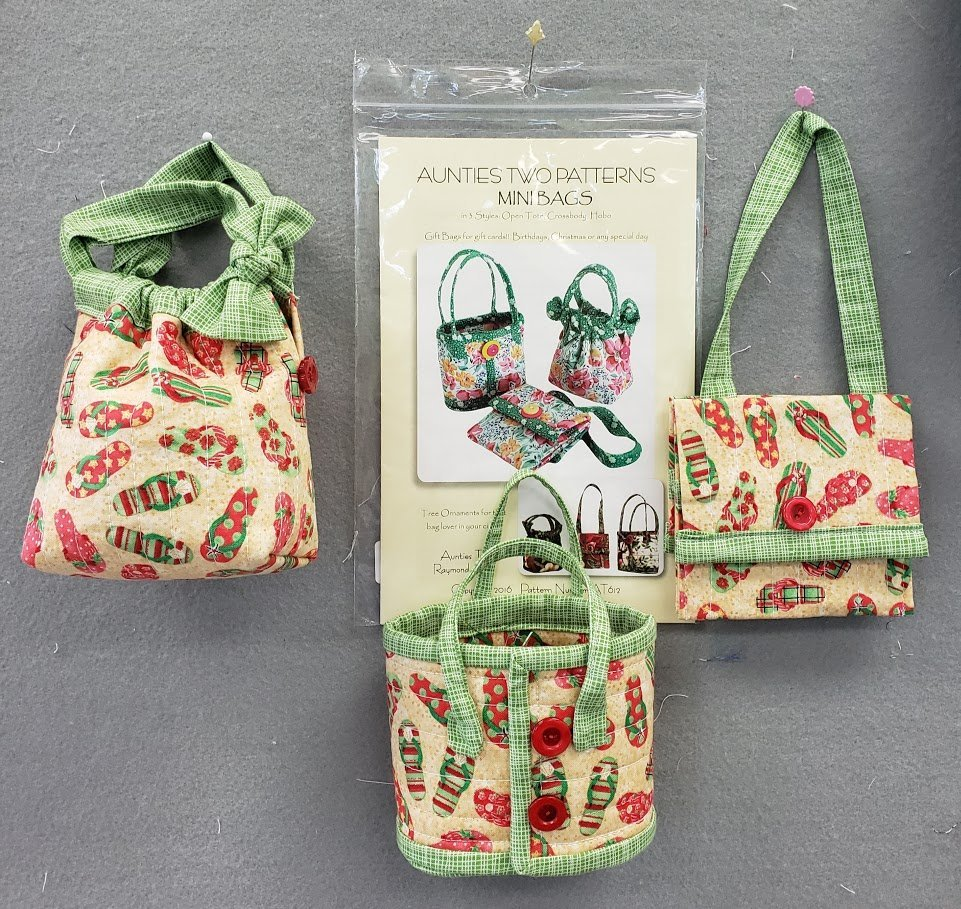# Mini Bags!

Fun cute mini bags in three styles: Open Tote, Hobo and Crossbody.
All approximately 4” x 4” great for gift card giving, perfect for Christmas tree ornaments or little girls first purse
Class is Tuesday, September 10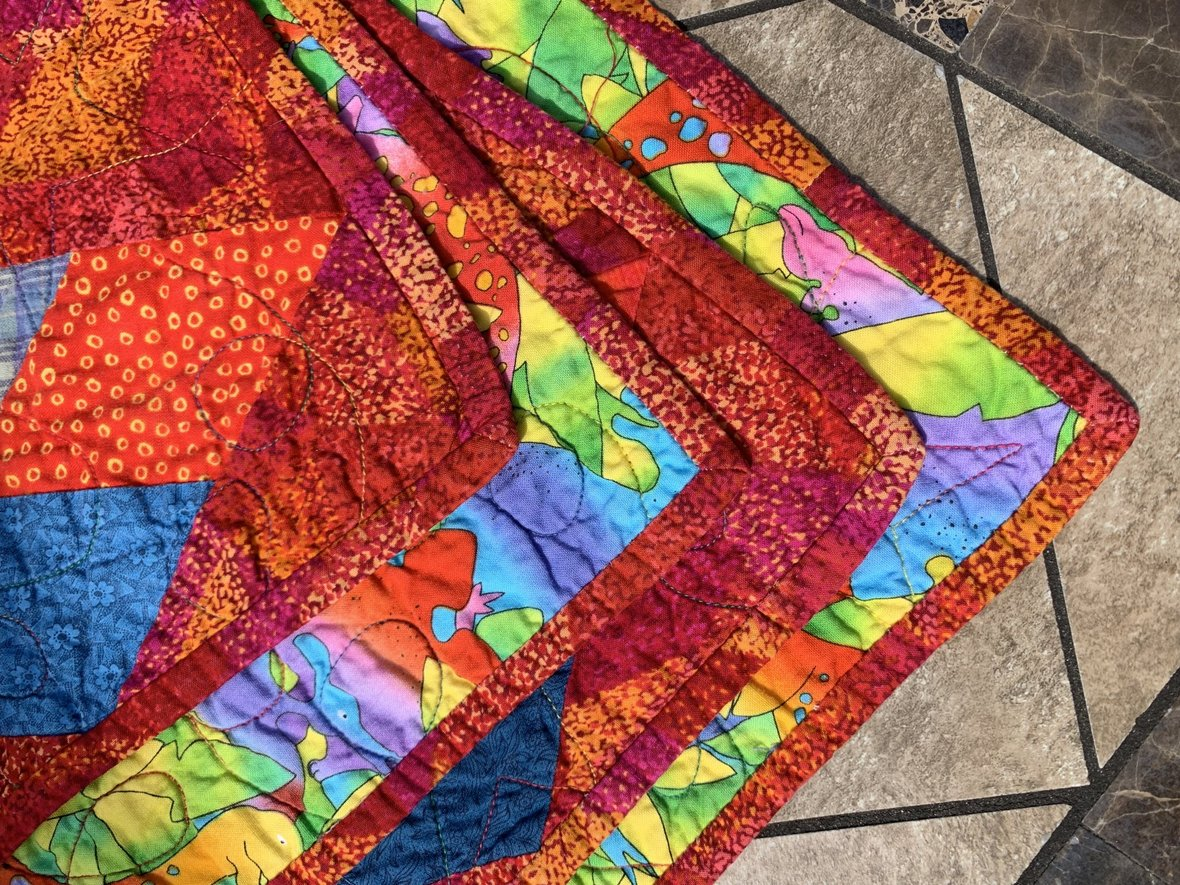# Brilliant Bindings

Learn the basics of finishing your quilt edges and applying professional looking binding.
This is a fun, quick, easy skill builder class, learning multiple techniques.
Class is Sunday, September 15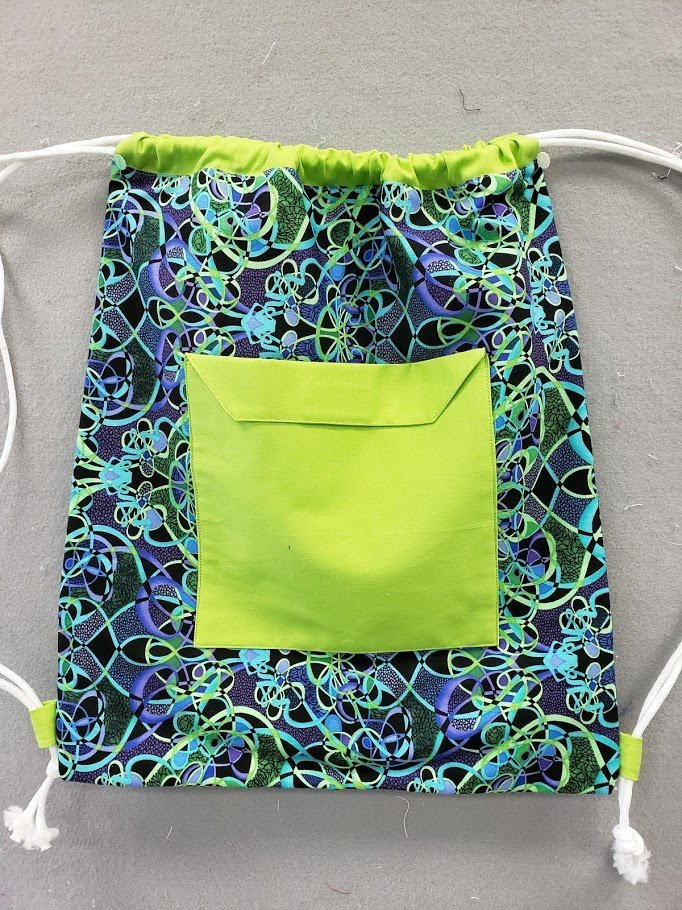# SewDays Backpack

==========
Easy drawstring backpack class designed for the new/inexperienced sewer.
Class is Monday, September 16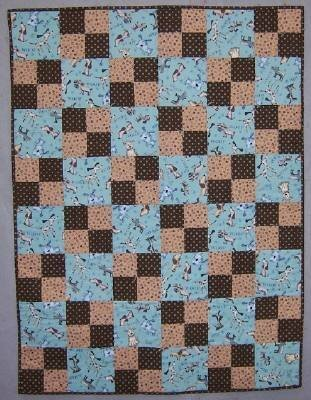# Beginning Quilt Making!

5 session class that takes you from start to finish!
Small class size insures individual attention.
Class starts Tuesday, September 17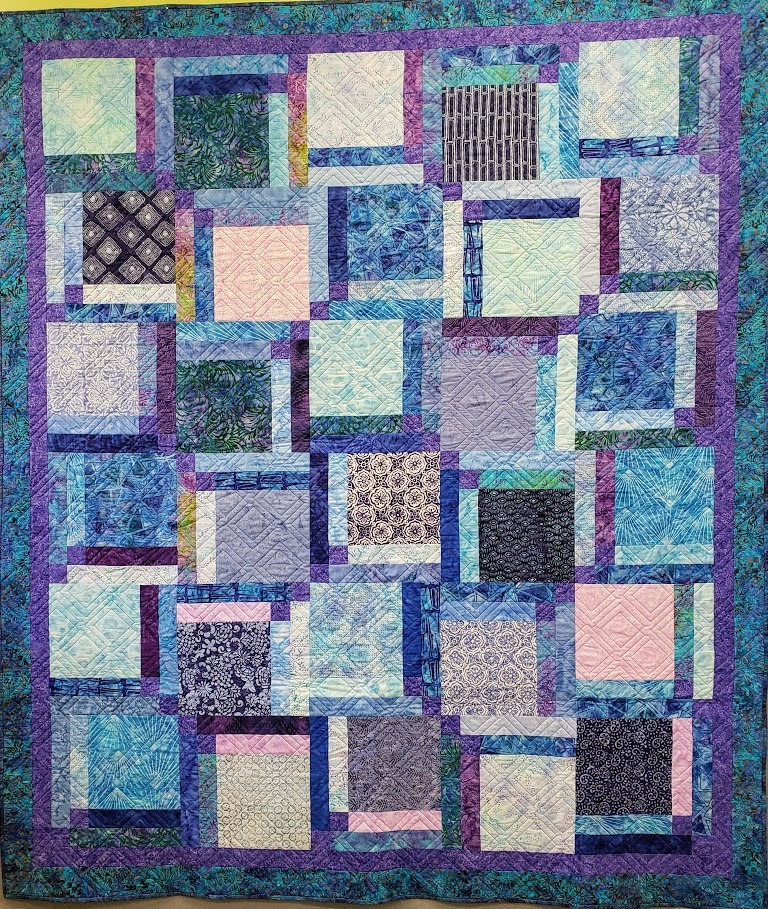# Cake Mix

Grab your favorite precuts or make your own and spend the day with Marlyn creating this big, beautiful quilt!
Class is Wednesday, September 18

# And there's more!

 table div table+table+table+table+table+table+table+table+table+table+table+table+table+table+table+table+table+table+table+table+table+table+table+table+table+table+table+table+table+table+table+table+table+table+table+table+table div table{width:100%;padding:0}table div table+table+table+table+table+table+table+table+table+table+table+table+table+table+table+table+table+table+table+table+table+table+table+table+table+table+table+table+table+table+table+table+table+table+table+table+table div table img{width:96.23%;padding:0;float:none}table div table+table+table+table+table+table+table+table+table+table+table+table+table+table+table+table+table+table+table+table+table+table+table+table+table+table+table+table+table+table+table+table+table+table+table+table+table div table td{width:100%;padding:0 1.88% 18px}/* styles */# FB Live is the place to be Sunday at 7pm!

You will be the 1st to see new fabrics, notions, kits and other great things that you need to know about!

There will be prizes, live demos and special pricing!

You will be able to purchase during the Live Broadcast and we can ship - sort of like Stuff Your Stocking but all in one night.

## If you miss the FB Live - not to worry!

You can view the replay on our FB Page AND you can still purchase at the special prices until 9pm Monday night!

We will go "live" at 6:55 and see what the hounds are up to, then
start the Soiree at 7pm (Eastern)

## Join me for a fun evening!

 table div table+table+table+table+table+table+table+table+table+table+table+table+table+table+table+table+table+table+table+table+table+table+table+table+table+table+table+table+table+table+table+table+table+table+table+table+table+table+table+table+table div table{width:100%;padding:0}table div table+table+table+table+table+table+table+table+table+table+table+table+table+table+table+table+table+table+table+table+table+table+table+table+table+table+table+table+table+table+table+table+table+table+table+table+table+table+table+table+table div table img{width:96.23%;padding:0;float:none}table div table+table+table+table+table+table+table+table+table+table+table+table+table+table+table+table+table+table+table+table+table+table+table+table+table+table+table+table+table+table+table+table+table+table+table+table+table+table+table+table+table div table td{width:100%;padding:0 1.88% 18px}/* styles */# Open 7 days a week for your convenience!

Monday through Saturday, 10am to 5pm
Sunday 12pm to 4pm

See you soon!

## Be sure to tune in to the Sunday Soiree on Facebook!

 table div table+table+table+table+table+table+table+table+table+table+table+table+table+table+table+table+table+table+table+table+table+table+table+table+table+table+table+table+table+table+table+table+table+table+table+table+table+table+table+table+table+table+table div table{width:100%;padding:0}table div table+table+table+table+table+table+table+table+table+table+table+table+table+table+table+table+table+table+table+table+table+table+table+table+table+table+table+table+table+table+table+table+table+table+table+table+table+table+table+table+table+table+table div table img{width:96.23%;padding:0;float:none}table div table+table+table+table+table+table+table+table+table+table+table+table+table+table+table+table+table+table+table+table+table+table+table+table+table+table+table+table+table+table+table+table+table+table+table+table+table+table+table+table+table+table+table div table td{width:100%;padding:0 1.88% 18px}/* styles *//* styles */ Whatcha doin' up on the table Stanley?
 table.module-44{width:72.64%;padding:0}table div table+table+table+table+table+table+table+table+table+table+table+table+table+table+table+table+table+table+table+table+table+table+table+table+table+table+table+table+table+table+table+table+table+table+table+table+table+table+table+table+table+table+table+table+table div table{width:72.64%;float:none;margin-left:auto;margin-right:auto;padding:0}table div table+table+table+table+table+table+table+table+table+table+table+table+table+table+table+table+table+table+table+table+table+table+table+table+table+table+table+table+table+table+table+table+table+table+table+table+table+table+table+table+table+table+table+table+table div table a{border:0 none;text-decoration:none}table div table+table+table+table+table+table+table+table+table+table+table+table+table+table+table+table+table+table+table+table+table+table+table+table+table+table+table+table+table+table+table+table+table+table+table+table+table+table+table+table+table+table+table+table+table div table img{width:100%!important;border:0 none;text-decoration:none}table div table+table+table+table+table+table+table+table+table+table+table+table+table+table+table+table+table+table+table+table+table+table+table+table+table+table+table+table+table+table+table+table+table+table+table+table+table+table+table+table+table+table+table+table+table div table td{width:100%;padding:0}/* styles */
 Like   Pin   +1